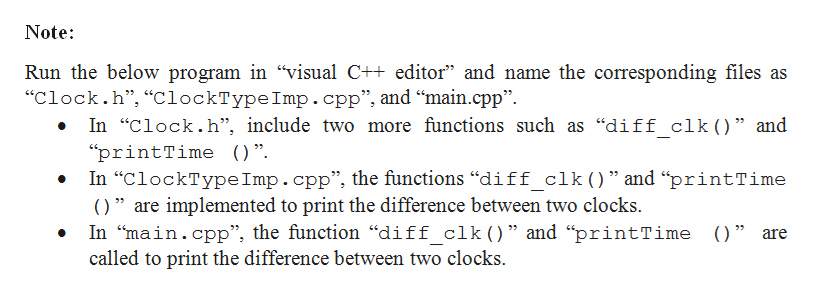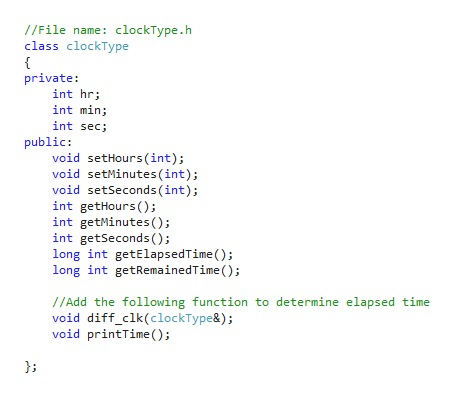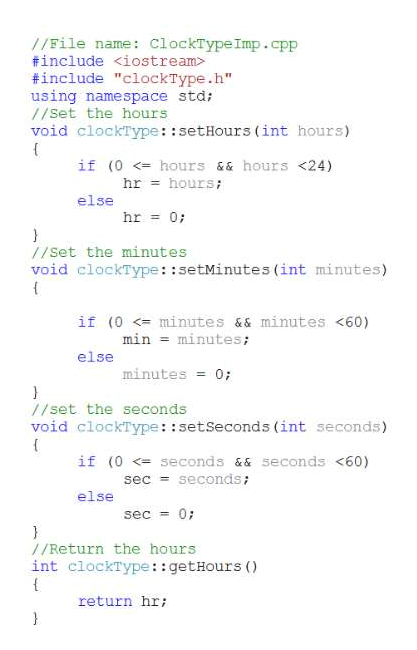I'm not sure how to make the clock show just seconds of the day and the remaining time with this problem.Enhance Programming Exercise 9 by adding functions to the class clockType so that a program that uses this class can perform the following operations:Returns the elapsed time of the day of a clock in seconds.Returns the remaining time of the dayof a clock in seconds.Determines and outputs how far apart in time two clocks are. Outputs the time in the form hr:min:sec. Also, write a program to test yourclass.   clockType.hclockTypeImp.cppmain.cpp     class clockType { public:    void setTime(int hours, int minutes, int seconds);    void setHours(int hours);    void setMinutes(int minutes);    void setSeconds(int seconds);     void getTime(int& hours, int& minutes, int& seconds) const;     int getElapsedTimeOfDay();    int getRemainingTimeOfDay(); ​    int getHours() const   {        return hr;   }     int getMinutes() const   {        return min;   }    int getSeconds() const   {        return sec;   }     void printTime() const;     void incrementSeconds();     void incrementMinutes();     void incrementHours();     bool equalTime(const clockType& otherClock) const;     clockType(int hours, int minutes, int seconds);     clockType();  private:    int hr;  //variable to store the hours    int min; //variable to store the minutes    int sec; //variable to store the seconds  };

Question

I'm not sure how to make the clock show just seconds of the day and the remaining time with this problem.

Enhance Programming Exercise 9 by adding functions to the class clockType so that a program that uses this class can perform the following operations:

• Returns the elapsed time of the day of a clock in seconds.
• Returns the remaining time of the day
• of a clock in seconds.
• Determines and outputs how far apart in time two clocks are. Outputs the time in the form hr:min:sec. Also, write a program to test yourclass.

• clockType.h
• clockTypeImp.cpp
• main.cpp
•

class clockType

{

public:

void setTime(int hours, int minutes, int seconds);

void setHours(int hours);

void setMinutes(int minutes);

void setSeconds(int seconds);

void getTime(int& hours, int& minutes, int& seconds) const;

int getElapsedTimeOfDay();

int getRemainingTimeOfDay();

int getHours() const

{

return hr;

}

int getMinutes() const

{

return min;

}

int getSeconds() const

{

return sec;

}

void printTime() const;

void incrementSeconds();

void incrementMinutes();

void incrementHours();

bool equalTime(const clockType& otherClock) const;

clockType(int hours, int minutes, int seconds);

clockType();

private:

int hr;  //variable to store the hours

int min; //variable to store the minutes

int sec; //variable to store the seconds

};

Step 1help_outlineImage TranscriptioncloseNote Run the below program in "visual C++ editor" and name the corresponding files as "Clock.h", "ClockTypeImp.cpp", and "main.cpp" In "Clock.h", include two more functions such as "diff clk() " and "printTime ) " In "ClockType Imp.cpp", the functions "diff_clk()" and "printTime |() " are implemented to print the difference between two clocks. In "main.cpp", the function "diff clk( ) " and "printTime )" are called to print the difference between two clocks. 27 fullscreen
Step 2help_outlineImage Transcriptionclose//File name: clockType.h class clockType { private: int hr int min int sec; public: void setHours (int); void setMinutes (int) void setSeconds (int) int getHours () int getMinutes ( ) int getSeconds () long int getElapsedTime ) long int getRemainedTime (); //Add the following function to determine elapsed time void diff_clk(clockType&); void printTime () fullscreen
Step 3help_outlineImage Transcriptionclose//File name: ClockType Imp.cpp finclude #include "clockType.h" using namespace std 1/set the hours void clockType::setHours (int hours) { if (0 hours && hours <24) hours hr else hr = 07 //Set the minutes void clockType: :setMinutes (int minutes) { if (0

Want to see the full answer?

See Solution

Want to see this answer and more?

Our solutions are written by experts, many with advanced degrees, and available 24/7

See Solution
Tagged in

Computer Science